# Introduction to continuous systems and fields

Certain mechanical issues require a continuous system. For instance, consider the issue of an elastic solid that is vibrating. Here, the oscillation is caused by each point of the continuous solid, and the full motion can only be described by stating the location coordinates of every point. We employ the following strategy to change the preceding formulation of mechanics: changing a discrete system to a continuous system.

The transition from a discrete to a continuous system

Consider an elastic rod that is infinitely long and capable of small longitudinal vibrations, or oscillatory displacement of the rod's particles parallel to the rod's axis. An infinite chain of points with equal masses, separated a distance apart, and connected by uniform massless springs with Boltz constant k forms a discrete particle system that resembles a continuous rod. Assume that the mass point can travel only along the chain's length. The discrete system will then be recognized as a linear polyatomic molecule extension. Therefore, using the standard method for small oscillations, we may obtain the equations characterizing the motion.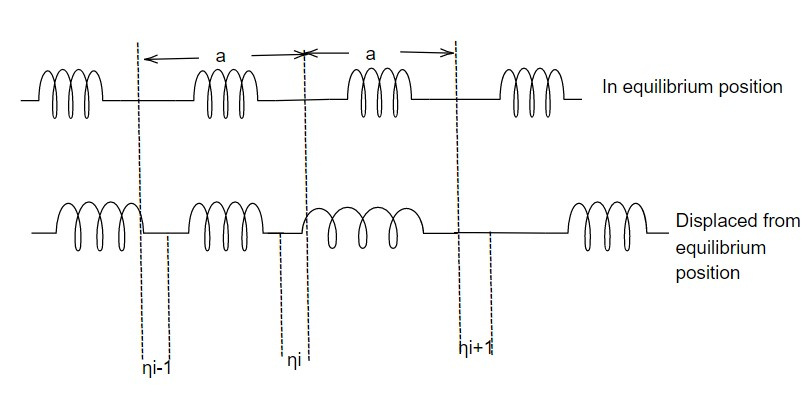Denoting the displacement of higher particle from its equilibrium position by ηi, then kinetic energy iswhere m is mass of each particle.

The corresponding potential energy is the sum of the potential energies of each spring as a result of being stretched or compressed from its equilibrium position. i.eNow the Lagrangian for the system (elastic rod) is given by

L = T- V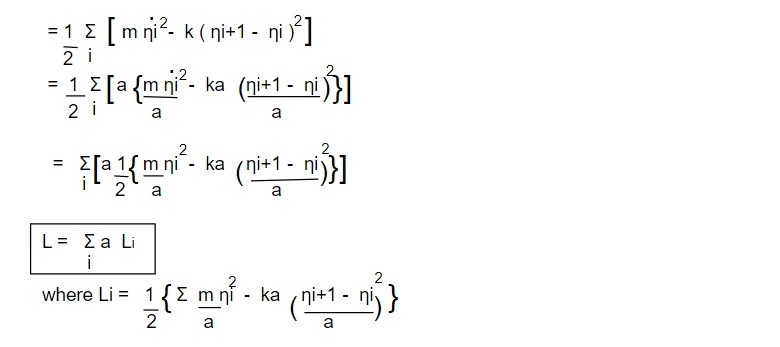The resulting Lagrange equation of motion for the coordinate ηi are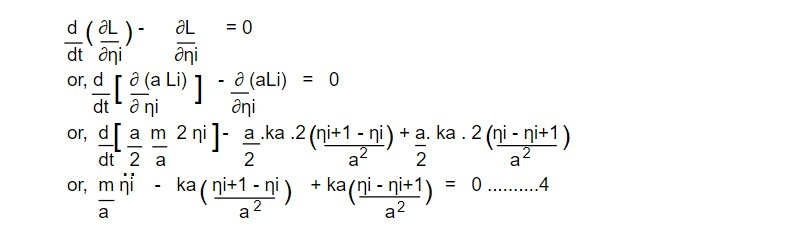It is clear that m/a reduced to μ, the mass per unit length of the continuous system (m/a = μ ). For an elastic rod obeying hook's law, we have

F ∝ ξ

F = Yξ ...............................5

where ξ is the elongation per unit length and Y is Young's modulus.

Now the extension of the length 'a' of a discrete system per unit length will be

ξ = (ηi+1 - ηi)/a

Then F= Y (ηi+1 - ηi)/a

= ka (ηi+1 - ηi)/a ........................6

On going from discrete to continuous system for case, the integer index 'i ' becomes the continuous partition coordinate x . i.e ηi = η(x)

Further the quantity

(ηi+1 - ηi)/a =[ η(x+a) - η(x)]/a

Taking the limit as 'a' playing the rate of dx approaches zero.Finally, the summation over a discrete number of particles become an integral over 'x '(the length of the rod). Then Lagrangian for continuous system becomes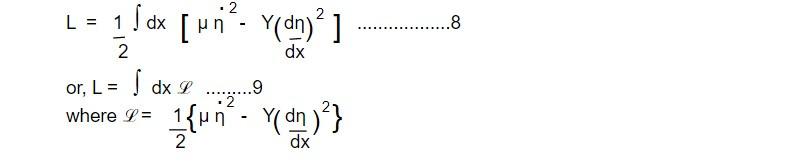is called the Lagrangian density for continuous system.

Hence equation of the motion for the continuous system (elastic rod) is given by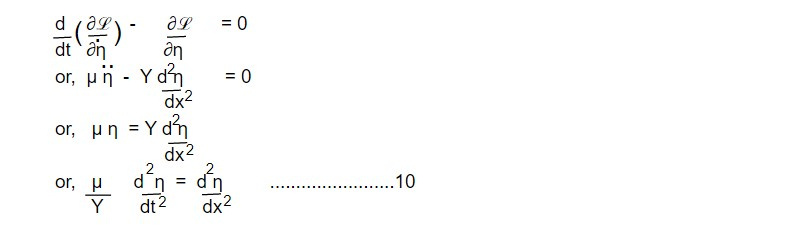Comparing equation 10 with familiar wave equation in 1 dimensional,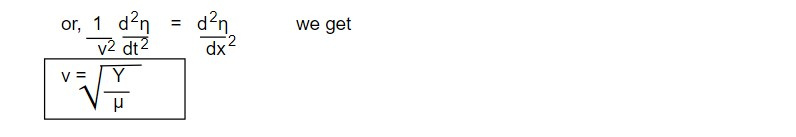which is the propagation velocity for the continuous system.

This note is taken from Statistical Mechanics, MSC physics, Nepal.

This note is a part of the Physics Repository.